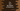# 3 different C++ program to find the largest of three numbers## 3 different C++ program to find the largest of three numbers:

This post will show you how to find the largest of three numbers in C++. Our program will take the numbers as inputs from the user and print out the result.

The program will:

• Take all three numbers input one by one
• Prints out the largest of these three

## Method 1: C++ program to find the largest of three:

``````#include <iostream>
using namespace std;

int main()
{
int firstNumber, secondNumber, thirdNumber;

cout << "Enter the first number :" << endl;
cin >> firstNumber;

cout << "Enter the second number :" << endl;
cin >> secondNumber;

cout << "Enter the third number :" << endl;
cin >> thirdNumber;

if (firstNumber > secondNumber)
{
if (thirdNumber > firstNumber)
{
cout << thirdNumber <<" is the largest" << endl;
}
else
{
cout << firstNumber <<" is the largest" << endl;
}
}
else
{
if (thirdNumber > secondNumber)
{
cout << thirdNumber <<" is the largest" << endl;
}
else
{
cout << secondNumber <<" is the largest" << endl;
}
}
}``````

### Explanation :

In this example,

• we are taking the numbers as input from the user and storing it in firstNumber, secondNumber and thirdNumber variables.
• Using nested if-else blocks, we are finding the largest.

### Sample Output :

``````Enter the first number :
3
Enter the second number :
2
Enter the third number :
1
3 is the largest``````

### Method 2: Using a different function to find the max of two numbers :

We can write one new function to find the max of two numbers and use that function to find the max of three.

Let’s take a look at the below program :

``````#include <iostream>
using namespace std;

int findMax(int first, int second)
{
return (first > second) ? first : second;
}

int main()
{
int firstNumber, secondNumber, thirdNumber;

cout << "Enter the first number :" << endl;
cin >> firstNumber;

cout << "Enter the second number :" << endl;
cin >> secondNumber;

cout << "Enter the third number :" << endl;
cin >> thirdNumber;

int max = findMax(firstNumber, findMax(secondNumber, thirdNumber));

cout << "Maximum number : " << max << endl;
}``````

### Explanation :

• Here, we have created one new function findMax to find the maximum of two integers.
• We are using findMax to find the maximum of secondNumber, thirdNumber and maximum of this result is compared with firstNumber to find the final maximum value.

This program will print the same output.

### Method 3: Using std::max :

std::max is another way to find the maximum of two values. We can use it instead of a different function to find out the max of three :

``````#include <iostream>

using namespace std;

int main()
{
int firstNumber, secondNumber, thirdNumber;

cout << "Enter the first number :" << endl;
cin >> firstNumber;

cout << "Enter the second number :" << endl;
cin >> secondNumber;

cout << "Enter the third number :" << endl;
cin >> thirdNumber;

int maxValue = std::max(firstNumber, std::max(secondNumber, thirdNumber));

cout << "Maximum number : " << maxValue << endl;
}``````

It will give the same result. But it is more concise that the other solutions.